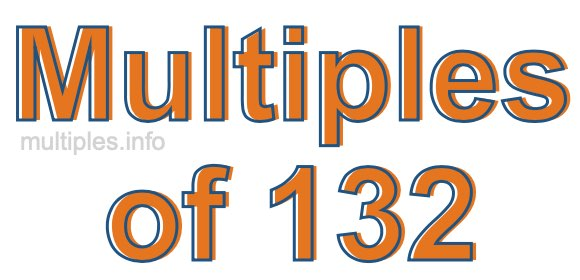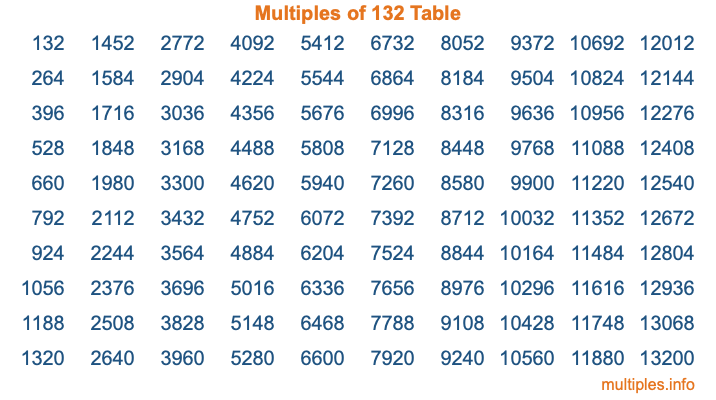Multiples of 132Welcome to the Multiples of 132 page. Here we will first teach you everything you will ever need to know about the multiples of 132, and then give you a study guide summary of everything we taught you to make sure you remember it all. Use this page to look up facts and learn information about the multiples of 132. This page will make you a multiples of one hundred thirty-two expert!

Definition of Multiples of 132
Multiples of 132 are all the numbers that when divided by 132 equal an integer. Each of the multiples of 132 are called a multiple. A multiple of 132 is created by multiplying 132 by an integer.

Therefore, to create a list of multiples of 132, you start with 1 multiplied by 132, then 2 multiplied by 132, then 3 multiplied by 132, and so on for as long as you want. Thus, the list of the first five multiples of 132 is 132, 264, 396, 528, and 660. To see a larger list of multiples of 132, see the printable image of Multiples of 132 further down on this page. We also have a category where you can choose any nth multiple of 132.

Multiples of 132 Checker
The Multiples of 132 Checker below checks to see if any number of your choice is a multiple of 132. In other words, it checks to see if there is any number (integer) that when multiplied by 132 will equal your number. To do that, we divide your number by 132. If the the quotient is an integer, then your number is a multiple of 132.

Is  a multiple of 132?

Least Common Multiple of 132 and ...
A Least Common Multiple (LCM) is the lowest multiple that two or more numbers have in common. This is also called the smallest common multiple or lowest common multiple and is useful to know when you are adding our subtracting fractions. Enter one or more numbers below (132 is already entered) to find the LCM.

Check out our LCM Calculator if you need more details about the Least Common Multiple or if you need the LCM for different numbers for adding and subtraction fractions.

nth Multiple of 132
As we stated above, 132 is the first multiple of 132, 264 is the second multiple of 132, 396 is the third multiple of 132, and so on. Enter a number below to find the nth multiple of 132.

th multiple of 132

Multiples of 132 vs Factors of 132
132 is a multiple of 132 and a factor of 132, but that is where the similarities end. All postive multiples of 132 are 132 or greater than 132. All positive factors of 132 are 132 or less than 132.

Below is the beginning list of multiples of 132 and the factors of 132 so you can compare:

Multiples of 132: 132, 264, 396, 528, 660, etc.

Factors of 132: 1, 2, 3, 4, 6, 11, 12, 22, 33, 44, 66, 132

As you can see, the multiples of 132 are all the numbers that you can divide by 132 to get a whole number. The factors of 132, on the other hand, are all the whole numbers that you can multiply by another whole number to get 132.

It's also interesting to note that if a number (x) is a factor of 132, then 132 will also be a multiple of that number (x).

Multiples of 132 vs Divisors of 132
The divisors of 132 are all the integers that 132 can be divided by evenly. Below is a list of the divisors of 132.

Divisors of 132: 1, 2, 3, 4, 6, 11, 12, 22, 33, 44, 66, 132

The interesting thing to note here is that if you take any multiple of 132 and divide it by a divisor of 132, you will see that the quotient is an integer.

Multiples of 132 Table
Below is an image of the first 100 multiples of 132 in a table. The table is in chronological order, column by column. The first column has the first ten multiples of 132, the second column has the next ten multiples of 132, and so on.The Multiples of 132 Table is also referred to as the 132 Times Table or Times Table of 132. You are welcome to print out our table for your studies.

Negative Multiples of 132
Although not often discussed or needed in math, it is worth mentioning that you can make a list of negative multiples of 132 by multiplying 132 by -1, then by -2, then by -3, and so on, to get the following list of negative multiples of 132:

-132, -264, -396, -528, -660, etc.

Multiples of 132 Summary
Below is a summary of important Multiples of 132 facts that we have discussed on this page. To retain the knowledge on this page, we recommend that you read through the summary and explain to yourself or a study partner why they hold true.

There are an infinite number of multiples of 132.

A multiple of 132 divided by 132 will equal a whole number.

132 divided by a factor of 132 equals a divisor of 132.

The nth multiple of 132 is n times 132.

The largest factor of 132 is equal to the first positive multiple of 132.

132 is a multiple of every factor of 132.

132 is a multiple of 132.

A multiple of 132 divided by a divisor of 132 equals an integer.

132 divided by a divisor of 132 equals a factor of 132.

Any integer times 132 will equal a multiple of 132.

Multiples of a Number
Here you can get the multiples of another number, all with the same attention to detail as we did for multiples of 132 on this page.

Multiples of
Multiples of 133
Did you find our page about multiples of one hundred thirty-two educational? Do you want more knowledge? Check out the multiples of the next number on our list!